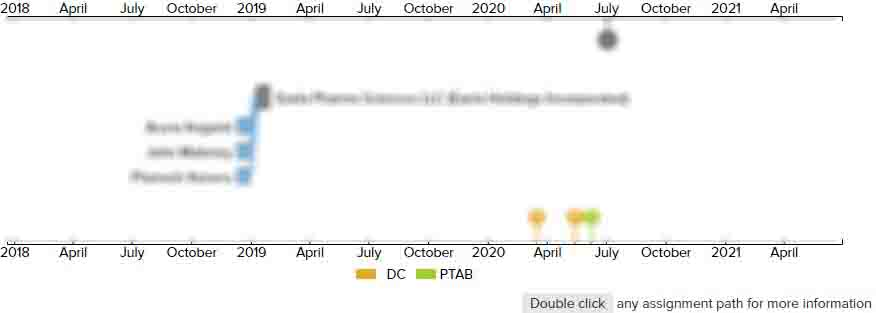# Common voltage feedback compensation circuit, method, and flat display device

• US 11,114,052 B2
• Filed: 09/29/2018
• Issued: 09/07/2021
• Est. Priority Date: 06/29/2018
• Status: Active Grant
##### First Claim
Patent Images

1. A common voltage feedback compensation circuit, comprising:

• a switch selecting circuit, wherein a feedback common voltage is inputted to a first end of the switch selecting circuit, a first reference voltage is inputted to a second end of the switch selecting circuit, and a second reference voltage is inputted to a third end of the switch selecting circuit, the switch selecting circuit is configured to determine a range of the feedback common voltage received from a display panel according to the first reference voltage and the second reference voltage, and an output end of the switch selecting circuit is configured to output corresponding switch signals according to a determining result;

a switch circuit, wherein the feedback common voltage is inputted to an input end of the switch circuit, an output end of the switch circuit connects to an input end of a compensation circuit, and the switch circuit is configured to be disconnected or closed according to the corresponding switch signals;

an attenuation circuit, wherein the feedback common voltage is inputted to an input end of the attenuation circuit, and an output end of the attenuation circuit connects to an input end of the compensation circuit;

when the switch circuit is disconnected, the feedback common voltage is inputted to and is attenuated by the attenuation circuit, and the attenuated feedback common voltage is outputted to the compensation circuit;

when the switch circuit is closed, the feedback common voltage is directly inputted to the compensation circuit;

the compensation circuit, wherein the input end of the compensation circuit connects to the output end of the switch circuit and the output end of the attenuation circuit, and an output end of the compensation circuit is configured to output common voltage compensation signals;

wherein the switch selecting circuit comprises;

a first comparator, wherein a non-inverting input end of the first comparator is configured to receive the feedback common voltage, an inverting input end of the first comparator is configured to receive a first reference voltage, an output end of the first comparator is configured to output a first comparison result to a logical processor according to a comparison result between the feedback common voltage and the first reference voltage;

a second comparator, wherein an inverting input end of the second comparator is configured to receive the feedback common voltage, a non-inverting input end of the second comparator is configured to receive a second reference voltage, the second comparator is configured to output a second comparison result to the logical processor according a comparison result between the feedback common voltage and the second reference voltage;

the logical processor, wherein a first input end of the logical processor is configured to receive the first comparison result, a second input end of the logical processor is configured to receive the second comparison result, the logical processor is configured determine the range of the feedback common voltage according to the first comparison result and the second comparison result, and an output end of the logical processor is configured to output the corresponding switch signals to the switch circuit.

• ##### 1 Assignment
Timeline View
Assignment View×
×